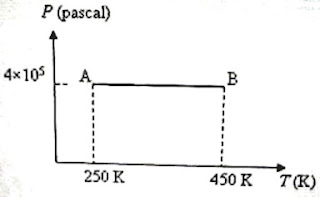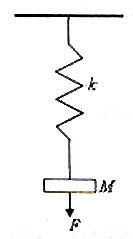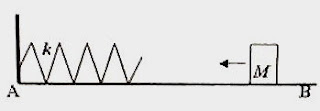## Pages

`“Life is like riding a bicycle.  To keep your balance you must keep moving.”–Albert Einstein`

## Tuesday, May 26, 2009

### AP Physics B – Multiple Choice Questions on Kinetic Theory and Thermodynamics

Everything that is really great and inspiring is created by the individual who can labour in freedom

– Albert Einstein

Equations to be remembered in kinetic theory of gases were discussed in the post dated 13th March 2008, followed by a discussion of some typical multiple choice questions (for practice) in the post dated 17th march 2008. You can access those posts by clicking on the label ‘kinetic theory’ below this post.

Important points related to thermodynamics were discussed in the post dated 21st May 2008, followed by a discussion of some typical multiple choice questions (for practice) in the post dated 26th May 2008. A couple of free response practice questions on thermodynamics were discussed in the post dated 3rd June 2008. You can access them by clicking on the label ‘thermodynamics’ below this post.

Today we will discuss a few more multiple choice questions on Kinetic Theory and Thermodynamics.

(1) A region of the earth’s atmosphere contains n molecules (treated as ideal gas molecules) per unit volume. The temperature of air in the region is T. If k represents Boltzmann’s constant and R represents universal gas constant, the pressure of air in the region is

(a) nT/k

(b) nkT

(c) RT/n

(d) nRT

(e) nRkT

Since PV = μRT where μ is the number of moles in the volume V of the gas at the pressure P and temperature T, we have

P = (μRT)/V

Since k = R/N where N is Avogadro number, R = Nk.

Therefore, P = (μNkT)/V.

But (μN)/V = n, the number of molecules per unit volume so that P = nkT

[The expression for the pressure P of a gas according to the kinetic theory is

P = (1/3) nmc2

The r.m.s.velocity c of the molecule is √(3kT/m) where m is the molecular mass. On substituting for c in the expression for P you can easily obtain the expression P = nkT].

(2) An ideal monoatomic gas is heated so that it expands at constant pressure. What percentage of the heat supplied to the gas is used to increase the internal energy of the gas?

(a) 100 %

(b) 0 %

(c) 60 %

(d) 50 %

(e) 40 %

Since a monoatomic gas molecule has 3 degrees of freedom, the molar heat capacity (also called molar specific heat) of the monoatomic gas at constant volume (CV) is (3/2)R where R is universal gas constant. Its molar heat capacity at constant pressure (CP) is (5/2)R . This follows from Meyer’s relation, CP = CV + R.

This means that when heat energy equal to (5/2)R joule is supplied to one mole of a monoatomic gas to make it expand at constant pressure, (3/2)R joule is used to increase the temperature by 1 K and the remaining R joule is used for doing work against the forces which oppose the expansion. 3/2 is 60 % of 5/2. So the correct option is (c).

(3) Oxygen and nitrogen in two enclosures have the same mass, volume and pressure. The ratio of the temperature of oxygen to that of nitrogen is

(a) 7/8

(b) 8/7

(c) 1

(d) 49/64

(e) 64/49

We have PV = μRT where μ is the number of moles in the volume V of the gas at the pressure P and temperature T.

Since P and V are the same for the two gases,

PV = μ1RT1 = μ2RT2 where μ1 and μ2 are the numbers of moles of oxygen and nitrogen and T1 and T2 are their temperatures respectively.

Therefore, μ1T1 = μ2T2 so that T1/T2 = μ2/μ1

The samples of the two gases have the same mass (say, m).

Therefore, μ1 = m/0.032 and μ2 = m/0.028 since the molar masses of oxygen and nitrogen are 0.032 kg and 0.028 kg respectively.

Therefore, T1/T2 = μ2/μ1 = 0.032/0.028 = 8/7.

(4) In a Carnot engine 7000 J of heat is absorbed from a source at 400 K and 4900 J of heat is rejected to the sink. The temperature of the sink is

(a) 200 K

(b) 220 K

(c) 260 K

(d) 280 K

(e) 340 K

We have Q1/T1 = Q2/T2 with usual notations.

Substituting the given values, 7000/400 = 4900/T2.

Therefore, T2 = (4900×400)/7000 = 280 K(5) Three moles of an ideal monoatomic gas is initially in the state A shown in the adjoining pressure-temperature graph. It is taken to state B without changing its pressure. If R is the universal gas constant, the work done by the gas in this process is

(a) 200 R

(b) 300 R

(c) 400 R

(d) 500 R

(e) 600 R

The work done by the gas in taking it from state A to state B = P∆V where ∆V is the increase in volume at constant pressure P.

We have PV = μRT where μ is the number of moles in the sample of the gas and R is the universal gas constant.

Therefore we have

P∆V = μR ∆T = 3×R(450 – 250) = 600R [Option (e)].

Find a few more multiple choice questions (with solution) on thermodynamics here.

## Tuesday, May 19, 2009

### AP Physics B&C - Work, Energy & Power - Multiple Choice Questions involving Springs

I am proud of the fact that I never invented weapons to kill

– Thomas Alva Edison

The essential points to be noted in the sections work, energy and power were discussed in the post dated 15th November 2008. Multiple choice questions in these sections were discussed in the posts dated 20th November 2008 and 30th November 2008. Two free response questions were discussed in the post dated 17th December 2008 You can access all those posts by clicking on the label ‘work’ or ‘energy’ below this post.

Today we will discuss a few multiple choice questions involving springs:(1) A spring of negligible mass and force constant (spring constant) k contained in a vertical barrel (Fig.) is free to move without friction vertically inside the barrel. A block of mass M, arranged at a height h from the top end of the unstretched spring, falls freely (without friction) from rest on the spring. The maximum compression x of the spring is given by the equation (acceleration due to gravity = g)

(a) mgx = ½ kx2

(b) mgh = ½ kx2

(c) mg (h+x) = ½ k[(h+x)2x2]

(d) mg (h+x) = ½ k[(h+x)2h2]

(e) mg (h+x) = ½ kx2

Since the mass M has moved down through a distance h+x when the spring undergoes the maximum compression x, the loss of gravitational potential energy by the mass M is mg (h+x).

The gain in the elastic potential energy by the spring in this condition is ½ kx2. The falling mass gains kinetic energy at the cost of gravitational potential energy. When it collides with the spring it transfers its kinetic energy to the spring and compresses the spring. When the spring is compressed to the maximum extent the entire kinetic energy of the mass is converted into elastic potential energy of the spring so that we have

mg (h+x) = ½ kx2

This equation gives the maximum compression x [Option (e)].

In the above question suppose the mass is initially resting on the spring. If the mass is depressed through a distance x and released, the spring will push the mass to a maximum height h (measured from the depressed position) given by the equation,mgh = ½ kx2

(2) A spring of negligible mass and of force constant (spring constant) 10 Nm–1 is suspended from the ceiling and it carries a mass M = 0.1 kg (Fig.). A downward force F is applied on this mass so that the spring suffers an additional stretch of 0.1 m. What is the work done by the force F? (Acceleration due to gravity, g = 10 ms–1).

(a) 0.14 J

(b) 0.15 J

(c) 0.2 J

(d) 0.39 J

(e) 0.1 J

The extension (x1) of the spring because of the suspended mass = Mg/k = (0.1×10)/10 = 0.1 m.

The work (W) done by the force F is equal to the increase in the total energy of the spring mass system. Note that the elastic potential energy of the spring has increased while the gravitational potential energy of the mass has decreased. Therefore we have

W = ½ k [(x1 + x2)2 x12] – mgx2

Or, W = ½ ×10×[0.04 – 0.01] – 0.01 = 0.14 J

(3) The elastic potential energy of a spring stretched by a small distance x is E. The spring is cut into two equal parts and one piece is stretched by the same distance x. The elastic potential energy of the stretched portion is

(a) E

(b) 2E

(c) 4E

(d) E/2

(e) E/4

When the length of a spring is halved, its spring constant is doubled. Its elastic potential energy for a given stretch x is quadrupled since the energy is directly proportional to the square of the stretch.

The correct option is (c).(4) The adjoining figure shows a block of mass M = 4 kg, moving with uniform velocity along a horizontal frictionless surface AB, towards a spring of force constant k = 100 Nm–1. One end of the spring is fixed to a rigid vertical wall. The block collides with the spring and produces in it a maximum compression of 0.1 m. What was the momentum of the block before the collision?

(a) 0.5 kg ms–1

(b) 2 kg ms–1

(c) 2.5 kg ms–1

(d) 4 kg ms–1

(e) 8 kg ms–1

Since the spring is compressed by 0.1 m, the elastic potential energy (which is ½ kx2 where x is the compression) of the spring is ½ ×100×(0.1)2 = 0.5 J.

Since the the block imparted this energy to the spring, the kinetic energy of the block was 0.5 J.

If p is the momentum of the block, we have

p2/2M = 0.5

Or, p2/(2×4) = 0.5 from which p = 2 kg ms–1

You can find similar useful questions (with solution) in this section here.

## Saturday, May 9, 2009

### AP Physics B&C- Multiple Choice Practice Questions on Circular Motion and Rotation

If we did all the things we are capable of, we would literally astound ourselves.

– Thomas Alva Edison

Normally you can expect questions on circular motion and rotation in the free response category and/or multiple choice category in the AP Physics Examination. The essential points to be remembered in circular motion and rotation were discussed in the post dated 20th January 2008. A free response question and a few multiple choice questions in this section were discussed afterwards. You can access all those posts by clicking on the label ‘rotation’ or ‘circular motion’ below this post.

Today we will discuss a few more multiple choice practice questions on circular motion and rotation:(1) A cubical wooden block of side L and mass M is placed on a rough horizontal surface. The friction between the block and the surface is sufficient so that the block can be toppled without sliding by applying a horizontal force on the block as shown in the adjoining figure. What is the minimum force F required for toppling the block?

(a) Mg

(b) 2 Mg

(c) Mg/2

(d) Mg/√2

The cube will topple by rotating about the edge P (Fig.). When the torque produced by the applied force F is just enough to topple the block, we can equate the toppling torque due to F to the restoring torque due to the weight Mg about the edge P:

FL = MgL/2

Therefore, F = Mg/2

(2) In uniform circular motion of a particle which one among the following does not remain constant?

(a) Kinetic energy

(b) Speed

(c) Angular momentum

(d) Momentum

(e) None

The momentum of the particle changes because of the change in its direction. [option (d)].(3) A simple pendulum of length L suspended from the ceiling is displaced and let go so that the bob of the pendulum moves with uniform angular velocity ω along a horizontal circle of radius R (Fig.). The bob has mass M and its size is negligible compared to R. The tension in the string of the pendulum is

(a) Mg

(b) MgL cos θ

(c) M (2 + g cos θ)

(d) M (R2ω4 + g2 cos2θ)1/2

(e) M (R2ω4 + g2)1/2

(This question is meant for AP Physics C aspirants. But AP Physics B aspirants too will ‘enjoy’ it).

There are three forces acting on the bob:

(i) The weight Mg of the bob acting vertically downwards.

(ii) The centrifugal force MRω2 acting radially outwards.

(iii) The tension T in the string.

Evidently the tension T is the resultant of the other two forces so that we have

T = [(MRω2)2 + (Mg)2]1/2

Thus T = M (R2ω4 + g2)1/2

(4) For AP Physics C

The moment of inertia of a thin uniform circular ring of mass M and radius R about an axis passing through the edge and parallel to the plane of the ring is

(a) 2 MR2

(b) MR2

(c) 3MR2/2

(d) MR2/4

(e) 3MR2/4

Since the moment of inertia of a thin ring about the central axis passing perpendicular to the plane of the ring is MR2, its moment of inertia about any diameter is MR2/2. (This follows from the perpendicular axes theorem).

The moment of inertia about an axis passing through the edge and parallel to the plane of the ring (as obtained by applying the parallel axis theorem) is MR2/2 + MR2 = 3MR2/2.

You will find a few more multiple choice questions (with solution) in this section here.